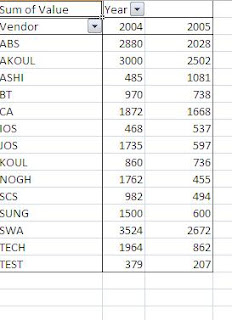## Saturday, July 9, 2011

### Create a simple pivot table using vba and hide grand total

If you want to create a new pivot table using vba and after creating a table you want to hide the grand total of columns and rows.

For example

Your Source data look likes belowand you want to create pivot table like belowHere is the code-

Sub simple_pivot_table()
Application.ScreenUpdating = False

' delete sheet Pivot Table 1 if preent in workbook
On Error Resume Next

Sheets("Pivot Table 1").Delete
ActiveSheet.Name = "Pivot Table 1"

Sheets("Data").Select
' selecting the source data in sheet name data
Sheets("Data").Range("a1:d" & Sheets("Data").Range("a65356").End(xlUp).Row)).CreatePivotTable TableDestination:=Sheets("Pivot Table 1").Cells(1, 1), _
TableName:="PivotTable1"
Sheets("Pivot Table 1").Select
With ActiveSheet.PivotTables("PivotTable1").PivotFields("Vendor")
.Orientation = xlRowField
.Position = 1
End With

With ActiveSheet.PivotTables("PivotTable1").PivotFields("Value")
.Orientation = xlColumnField
.Position = 1
End With
"PivotTable1").PivotFields("Value"), "Sum of Value", xlSum
With ActiveSheet.PivotTables("PivotTable1").PivotFields("Year")
.Orientation = xlColumnField
.Position = 1
End With
' chnage it to true if you want to show grand total
ActiveSheet.PivotTables("PivotTable1").RowGrand = False
' chnage it to true if you want to show grand total
ActiveSheet.PivotTables("PivotTable1").ColumnGrand = False

'hide field list window
ActiveWorkbook.ShowPivotTableFieldList = False
Application.ScreenUpdating = True

End Sub

Excel Macro File http://www.filefactory.com/file/cc4e537/n/pivot_tables_in_vba.xlsm

1.Hi i am unble to add one more column field in the above code. Kindly help me on this. I have use same code which matches my requirement exactly.

2.@ ragahva can you share the sample workbook.

Try something like this